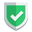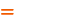我们相信：世界是美好的，你是我也是。平行空间的世界里面，不同版本的生活也在继续...

## 标的变量

``````import numpy as np
sn  = np.array([["苏", "南"], ["大", "叔"]])
sn2 = np.array([["苏", "大"], ["南", "叔"]])
sn3 = sn.T``````

## 传统双等号判断失败【不推荐】

``````print( sn==sn2 )
print( type(sn==sn2) )``````

``````[[ True False]
[False  True]]
<class 'numpy.ndarray'>``````

``````if (sn == sn2) :
print("ok")``````

``ValueError: The truth value of an array with more than one element is ambiguous. Use a.any() or a.all()``

## 方案一：(s==n).all()【不推荐】

``````print( (sn == sn2).all() )     # False
print( (sn == sn3).all() )     # False
print( (sn2 == sn3).all() )    # True``````

``````False
False
True``````

``````import numpy as np
s = np.array([[1,2,3]])
n = np.array([1,2,3])
print( (s == n).all() )        # True
print( np.array_equal(s, n) )  # False``````

## 方案二：np.array_equal(s,n)【推荐】

``````print( np.array_equal(sn, sn2) )     # False
print( np.array_equal(sn, sn3) )     # False
print( np.array_equal(sn2, sn3) )    # True``````

``````False
False
True``````

## 特殊方案：是否是本体

``````import numpy as np
sn = np.array([["苏", "南"], ["大", "叔"]])
sn2 = np.array([["苏", "大"], ["南", "叔"]])
sn3 = sn.T           # 这个也是两个变量哦
sn4 = sn3
sn5 = sn3.copy()

print(sn is sn2)     # False
print(sn is sn3)     # False
print(sn2 is sn3)    # False
print(sn3 is sn.T)   # False
print(sn3 is sn4)    # True
print(sn3 is sn5)    # False``````

## 结束语如果本文对您有帮助，或者节约了您的时间，欢迎打赏瓶饮料，建立下友谊关系。本博客不欢迎：各种镜像采集行为。请尊重原创文章内容，转载请保留作者链接。【福利】 腾讯云最新爆款活动！1核2G云服务器首年50元！【源码】本文代码片段及相关软件，请点此获取更多信息【绝密】秘籍文章入口，仅传授于有缘之人python